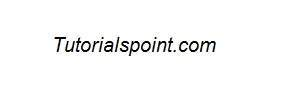# Java DIP - Applying Watermark

In this chapter we learn two ways of applying watermark on images. These ways are −

• Applying Text Watermark
• Applying Image watermark

## Applying Text Watermark

We use OpenCV function putText to apply text watermark to image. It can be found under Core package. Its syntax is given below −

```Core.putText(source, Text, Point, fontFace ,fontScale , color);
```

The parameters of this function are described below −

Sr.No. Parameter & Description
1

Source

It is source image.

2

Text

It is the string text that would appear on the image.

3

Point

It is the point where text should appear on image.

4

fontFace

Font type. For example − FONT_HERSHEY_SIMPLEX, FONT_HERSHEY_PLAIN, FONT_HERSHEY_COMPLEX etc.

5

fontScale

It is font scale factor that is multiplied by the font-specific base size.

6

color

It is text color.

Apart from the putText method, there are other methods provided by the Core class. They are described briefly −

Sr.No. Method & Description
1

normalize(Mat src, Mat dst, double alpha, double beta, int norm_type)

It normalizes the norm or value range of an array.

2

perspectiveTransform(Mat src, Mat dst, Mat m)

It performs the perspective matrix transformation of vectors.

3

phase(Mat x, Mat y, Mat angle)

It calculates the rotation angle of 2D vectors.

4

rectangle(Mat img, Point pt1, Point pt2, Scalar color)

It draws a simple, thick, or filled up-right rectangle.

5

reduce(Mat src, Mat dst, int dim, int rtype, int dtype)

It reduces a matrix to a vector.

6

transform(Mat src, Mat dst, Mat m)

It performs the matrix transformation of every array element.

### Example

The following example demonstrates the use of Core class to apply text watermark to an image −

```import org.opencv.core.Core;
import org.opencv.core.Mat;

import org.opencv.highgui.Highgui;
import org.opencv.imgproc.Imgproc;

public class Main {
public static void main( String[] args ) {

try{
Mat destination = new Mat(source.rows(),source.cols(), source.type());

Core.putText(source, "Tutorialspoint.com", new Point  (source.rows()/2,source.cols()/2), Core.FONT_ITALIC,new Double(1),new  Scalar(255));

Highgui.imwrite("watermarked.jpg", source);

} catch (Exception e) {
System.out.println("Error: "+e.getMessage());
}
}
}
```

### Output

When you execute the given code, the following output is seen −

#### Original Image#### Text Watermarked Image## Applying Image Watermark on Image

We are going to use OpenCV function addWeighted to apply image watermark to image. It can be found under Core package. Its syntax is given below −

```Core.addWeighted(InputArray src1, alpha, src2 (Watermark image), beta, gamma, OutputArray dst);
```

The parameters of this function are described below −

Sr.No. Parameter & Description
1

src1

It is first input array.

2

alpha

It is the weight of the first array elements.

3

src2

It is the second input array of the same size and channel number as src1.

4

beta

It is the weight of the second array elements.

5

gamma

It is the scalar added to each sum.

6

dst

It is the output array that has the same size and number of channels as the input arrays.

### Example

The following example demonstrates the use of Core class to apply image watermark to an image −

```import org.opencv.core.Core;
import org.opencv.core.Mat;

import org.opencv.highgui.Highgui;
import org.opencv.imgproc.Imgproc;

public class Main {
public static void main( String[] args ) {

try{

Rect ROI = new Rect(waterMark.rows() * 4,waterMark.cols(),  waterMark.cols(),waterMark.rows());

Core.addWeighted(source.submat(ROI), 0.8, waterMark, 0.2, 1,  source.submat(ROI));
Highgui.imwrite("watermarkedImage.jpg", source);

} catch (Exception e) {
System.out.println("Error: " + e.getMessage());
}
}
}
```

### Output

When you execute the given code, the following output is seen −

#### Original Image#### The Watermark Image#### Watermarked Image